# 1077 functions are available on our servers and libraries

This is a list of examples and functions which our servers provide. For details, see manuals of each system.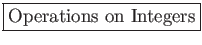idiv,irem (division with remainder), ishift (bit shifting), iand,ior,ixor (logical operations), igcd,(GCD by various methods such as Euclid's algorithm and the accelerated GCD algorithm), fac (factorial), inv (inverse modulo an integer), random (random number generator by the Mersenne twister algorithm).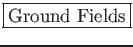Arithmetics on various fields: the rationals,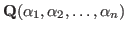(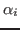is algebraic over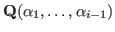),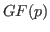(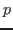is a prime of arbitrary size),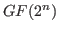.sdiv , srem (division with remainder), ptozp (removal of the integer content), diff (differentiation), gcd (GCD over the rationals), res (resultant), subst (substitution), umul (fast multiplication of dense univariate polynomials by a hybrid method with Karatsuba and FFT+Chinese remainder), urembymul_precomp (fast dense univariate polynomial division with remainder by the fast multiplication and the precomputed inverse of a divisor),fctr (factorization over the rationals), fctr_ff (univariate factorization over finite fields), af (univariate factorization over algebraic number fields), sp (splitting field computation).dp_gr_main (Groebner basis computation of a polynomial ideal over the rationals by the trace lifting), dp_gr_mod_main (Groebner basis over small finite fields), tolex (Modular change of ordering for a zero-dimensional ideal), tolex_gsl (Modular rational univariate representation for a zero-dimensional ideal), dp_f4_main (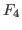over the rationals), dp_f4_mod_main (over small finite fields).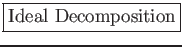primedec (Prime decomposition of the radical), primadec (Primary decomposition of ideals by Shimoyama/Yokoyama algorithm).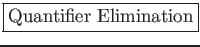qe (real quantifier elimination in a linear and quadratic first-order formula), simpl (heuristic simplification of a first-order formula).

 MTP2 = ex([x11,x12,x13,x21,x22,x23,x31,x32,x33],
x11+x12+x13 @== a1 @&& x21+x22+x23 @== a2 @&& x31+x32+x33 @== a3
@&& x11+x21+x31 @== b1 @&& x12+x22+x32 @== b2 @&& x13+x23+x33 @== b3
@&& 0 @<= x11 @&& 0 @<= x12 @&& 0 @<= x13 @&& 0 @<= x21
@&& 0 @<= x22 @&& 0 @<= x23 @&& 0 @<= x31 @&& 0 @<= x32 @&& 0 @<= x33)$ TSOL= a1+a2+a3@=b1+b2+b3 @&& a1@>=0 @&& a2@>=0 @&& a3@>=0 @&& b1@>=0 @&& b2@>=0 @&& b3@>=0$
 QE_MTP2 = qe(MTP2)\$
 qe(all([a1,a2,a3,b1,b2,b3],QE_MTP2 @equiv TSOL));
@trueplot (plotting of a univariate function), ifplot (plotting zeros of a bivariate polynomial), conplot (contour plotting of a bivariate polynomial function).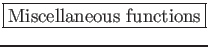det (determinant), qsort (sorting of an array by the quick sort algorithm), eval (evaluation of a formula containing transcendental functions such as sin, cos, tan, exp, log) roots (finding all roots of a univariate polynomial), lll (computation of an LLL-reduced basis of a lattice).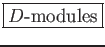(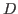is the Weyl algebra)

gb (Gröbner basis), syz (syzygy), annfs (Annhilating ideal of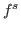), bfunction, schreyer (free resolution by the Schreyer method), vMinRes (V-minimal free resolution), characteristic (Characteristic variety), restriction in the derived category of-modules, integration in the derived category, tensor in the derived category, dual (Dual as a D-module), slope.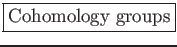deRham (The de Rham cohomology groups of, ext (Ext modules for a holonomic-moduleand the ring of formal power series).Helping to derive and prove combinatorial and special function identities, gkz (GKZ hypergeometric differential equations), appell (Appell's hypergeometric differential equations), indicial (indicial equations), rank (Holonomic rank), rrank (Holonomic rank of regular holonomic systems), dsolv (series solutions of holonomic systems).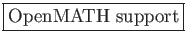om_xml (CMO to OpenMATH XML), om_xml_to_cmo (OpenMATH XML to CMO).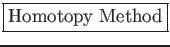phc (Solving systems of algebraic equations by numerical and polyhedral homotopy methods).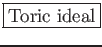tigers (Enumerate all Gröbner basis of a toric ideal. Finding test sets for integer program), arithDeg (Arithmetic degree of a monomial ideal), stdPair (Standard pair decomposition of a monomial ideal).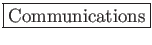ox_launch (starting a server), ox_launch_nox, ox_shutdown, ox_launch_generic, generate_port, try_bind_listen, try_connect, try_accept, register_server, ox_rpc, ox_cmo_rpc, ox_execute_string, ox_reset (reset the server), ox_intr, register_handler, ox_push_cmo, ox_push_local, ox_pop_cmo, ox_pop_local, ox_push_cmd, ox_sync, ox_get, ox_pops, ox_select, ox_flush, ox_get_serverinfo

In addition to these functions, Mathematica functions can be called as server functions.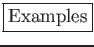sm1_deRham([x^3-y^2*z^2,[x,y,z]]);
[1,1,0,0]
/* dim H^i = 1 (i=0,1), =0 (i=2,3) */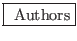Castro-Jiménez, Dolzmann, Hubert, Murao, Noro, Oaku, Okutani, Shimoyama, Sturm, Takayama, Tamura, Verschelde, Yokoyama.

Nobuki Takayama 2017-03-30